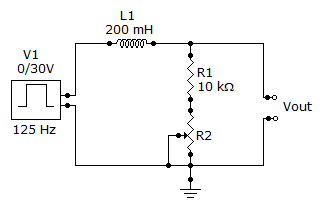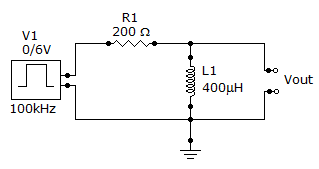# Electrical Engineering - Time Response of Reactive Circuits

Exercise : Time Response of Reactive Circuits - General Questions
6.
Referring this circuit, determine the maximum output voltage when a single pulse is applied as shown. The total resistance is 60.2.73 V
27.33 V
30 V
2.67 V
Explanation:
No answer description is available. Let's discuss.

7.
A steady-state condition is reached when
the output voltage reaches the average value of the input voltage
the output voltage reaches the input voltage
the output voltage reaches approximately 63% of the input voltage
the output voltage reaches the effective value of the input voltage
Explanation:
No answer description is available. Let's discuss.

8.
Referring to the give circuit, the output willdecay to zero at the end of the pulse
reach 6 V at the end of the pulse
reach 3.78 V at the end of the pulse
reach 5.16 V at the end of the pulse
Explanation:
No answer description is available. Let's discuss.

9.
A single 6 V pulse with a width of 600s is applied to an integrator consisting of a 150 kresistor and a 0.002F capacitor. To what voltage will the capacitor charge?
0 V
3.78 V
5.16 V
6 V